$$\newcommand{\id}{\mathrm{id}}$$ $$\newcommand{\Span}{\mathrm{span}}$$ $$\newcommand{\kernel}{\mathrm{null}\,}$$ $$\newcommand{\range}{\mathrm{range}\,}$$ $$\newcommand{\RealPart}{\mathrm{Re}}$$ $$\newcommand{\ImaginaryPart}{\mathrm{Im}}$$ $$\newcommand{\Argument}{\mathrm{Arg}}$$ $$\newcommand{\norm}{\| #1 \|}$$ $$\newcommand{\inner}{\langle #1, #2 \rangle}$$ $$\newcommand{\Span}{\mathrm{span}}$$

2.1: Relations, Graphs, and Functions

$$\newcommand{\vecs}{\overset { \rightharpoonup} {\mathbf{#1}} }$$

$$\newcommand{\vecd}{\overset{-\!-\!\rightharpoonup}{\vphantom{a}\smash {#1}}}$$

Skills to Develop

• State the domain and range of a relation.
• Identify a function.
• Use function notation

Graphs, Relations, Domain, and Range

The rectangular coordinate system1 consists of two real number lines that intersect at a right angle. The horizontal number line is called the x-axis2, and the vertical number line is called the y-axis3. These two number lines define a flat surface called a plane4, and each point on this plane is associated with an ordered pair5 of real numbers $$(x, y)$$. The first number is called the $$x$$-coordinate, and the second number is called the $$y$$-coordinate. The intersection of the two axes is known as the origin6, which corresponds sto the point $$(0, 0)$$.

The $$x$$- and $$y$$-axes break the plane into four regions called quadrants7, named using roman numerals I, II, III, and IV, as pictured. The ordered pair $$(x, y)$$ represents the position of points relative to the origin. For example, the ordered pair $$(−4, 3)$$ represents the position $$4$$ units to the left of the origin, and $$3$$ units above in the second quadrant.Figure 2.1.1

This system is often called the Cartesian coordinate system8, named after the French mathematician René Descartes (1596–1650).Figure 2.1.2

Next, we define a relation9 as any set of ordered pairs. In the context of algebra, the relations of interest are sets of ordered pairs $$(x, y)$$ in the rectangular coordinate plane. Typically, the coordinates are related by a rule expressed using an algebraic equation. For example, both the algebraic equations $$y = |x| − 2$$ and $$x = |y| + 1$$ define relationships between $$x$$ and $$y$$. Following are some integers that satisfy both equations:Figure 2.1.3

Here two relations consisting of seven ordered pair solutions are obtained:

$$y=|x|−2 has solutions \{(−3,1),(−2,0),(−1,−1),(0,−2),(1,−1),(2,0),(3,1)\}$$

$$and$$

$$x=|y|+1 has solutions \{(4,−3),(3,−2),(2,−1),(1,0),(2,1),(3,2),(4,3)\}$$

We can visually display any relation of this type on a coordinate plane by plotting the points.Figure 2.1.4

The solution sets of each equation will form a relation consisting of infinitely many ordered pairs. We can use the given ordered pair solutions to estimate all of the other ordered pairs by drawing a line through the given points. Here we put an arrow on the ends of our lines to indicate that this set of ordered pairs continues without bounds.Figure 2.1.5

The representation of a relation on a rectangular coordinate plane, as illustrated above, is called a graph10. Any curve graphed on a rectangular coordinate plane represents a set of ordered pairs and thus defines a relation.

The set consisting of all of the first components of a relation, in this case the x-values, is called the domain11. And the set consisting of all second components of a relation, in this case the y-values, is called the range12 (or codomain13). Often, we can determine the domain and range of a relation if we are given its graph.Figure 2.1.6

Here we can see that the graph of $$y=|x|−2$$ has a domain consisting of all real numbers, $$ℝ=(−∞,∞)$$, and a range of all y-values greater than or equal to $$−2, [−2,∞)$$. The domain of the graph of $$x=|y|+1$$ consists of all x-values greater than or equal to $$1, [1,∞)$$, and the range consists of all real numbers, $$ℝ=(−∞,∞)$$.

Example $$\PageIndex{1}$$:

Determine the domain and range of the following relation:Figure 2.1.7

Solution:

The minimum $$x$$-value represented on the graph is $$−8$$ all others are larger. Therefore, the domain consists of all $$x$$-values in the interval $$[−8,∞)$$. The minimum $$y$$-value represented on the graph is $$0$$; thus, the range is $$[0,∞)$$.Figure 2.1.8

Domain: $$[−8,∞)$$; range: $$[0,∞)$$

Functions

Of special interest are relations where every $$x$$-value corresponds to exactly one $$y$$-value. A relation with this property is called a function14.

Example $$\PageIndex{2}$$:

Determine the domain and range of the following relation and state whether it is a function or not: $$\{(−1, 4), (0, 7), (2, 3), (3, 3), (4, −2)\}$$

Solution:

Here we separate the domain (x-values), and the range (y-values), and depict the correspondence between the values with arrows.Figure 2.1.9

The relation is a function because each x-value corresponds to exactly one y-value.

The domain is $$\{−1, 0, 2, 3, 4\}$$ and the range is $$\{−2, 3, 4, 7\}$$. The relation is a function.

Example $$\PageIndex{3}$$:

Determine the domain and range of the following relation and state whether it is a function or not: $$\{(−4, −3), (−2, 6), (0, 3), (3, 5), (3, 7)\}$$

Solution:Figure 2.1.10

The given relation is not a function because the $$x$$-value $$3$$ corresponds to two $$y$$-values. We can also recognize functions as relations where no $$x$$-values are repeated.

The domain is $$\{−4, −2, 0, 3\}$$ and the range is $$\{−3, 3, 5, 6, 7\}$$. This relation is not a function.

Consider the relations consisting of the seven ordered pair solutions to $$y = |x| − 2$$and $$x = |y| + 1$$. The correspondence between the domain and range of each can be pictured as follows:Figure 2.1.11

Notice that every element in the domain of the solution set of $$y = |x| − 2$$ corresponds to only one element in the range; it is a function. The solutions to $$x = |y| + 1$$, on the other hand, have values in the domain that correspond to two elements in the range. In particular, the x-value $$4$$ corresponds to two y-values $$−3$$ and $$3$$. Therefore, $$x = |y| + 1$$ does not define a function.

We can visually identify functions by their graphs using the vertical line test15. If any vertical line intersects the graph more than once, then the graph does not represent a function.Figure 2.1.12

The vertical line represents a value in the domain, and the number of intersections with the graph represent the number of values to which it corresponds. As we can see, any vertical line will intersect the graph of $$y=|x|−2$$ only once; therefore, it is a function. A vertical line can cross the graph of $$x=|y|+1$$ more than once; therefore, it is not a function. As pictured, the $$x$$-value $$3$$ corresponds to more than one $$y$$-value.

Example $$\PageIndex{1}$$:

Given the graph, state the domain and range and determine whether or not it represents a function:Figure 2.1.13

Solution:

From the graph we can see that the minimum $$x$$-value is $$−1$$ and the maximum $$x$$-value is $$5$$. Hence, the domain consists of all the real numbers in the set from $$[−1,5]$$. The maximum $$y$$-value is $$3$$ and the minimum is $$−3$$; hence, the range consists of $$y$$-values in the interval $$[−3,3]$$.Figure 2.1.14

In addition, since we can find a vertical line that intersects the graph more than once, we conclude that the graph is not a function. There are many $$x$$-values in the domain that correspond to two $$y$$-values.

Domain: $$[−1,5]$$; range: $$[−3,3]$$; function: no

Exercise $$\PageIndex{1}$$

Given the graph, determine the domain and range and state whether or not it is a function:Figure 2.1.15

Domain: $$(−∞,15]$$; range:$$ℝ$$; function: no

Function Notation

With the definition of a function comes special notation. If we consider each x-value to be the input that produces exactly one output, then we can use function notation16:

$$f ( x ) = y$$

The notation $$f(x)$$ reads, “f of x” and should not be confused with multiplication. Algebra frequently involves functions, and so the notation becomes useful when performing common tasks. Here $$f$$ is the function name, and $$f (x)$$ denotes the value in the range associated with the value x in the domain. Functions are often named with different letters; some common names for functions are $$f, g, h, C$$, and $$R$$. We have determined that the set of solutions to $$y = |x| − 2$$ is a function; therefore, using function notation we can write:

\begin{aligned} y & = | x | - 2 \\ \color{Cerulean}{\downarrow} & \\ f ( x ) & = | x | - 2 \end{aligned}

It is important to note that $$y$$ and $$f(x)$$ are used interchangeably. This notation is used as follows:

$$\begin{array} { l } { f ( x )\:\: =\:\:\: | x | - 2 } \\ { \:\:\:\:\:\downarrow \quad\quad\quad \downarrow } \\ { f ( \color{Cerulean}{- 5}\color{Black}{ )} = | \color{Cerulean}{- 5}\color{Black}{ |} - 2 = 5 - 2 = 3 } \end{array}$$

Here the compact notation $$f(−5) = 3$$ indicates that where $$x = −5$$ (the input), the function results in $$y = 3$$ (the output). In other words, replace the variable with the value given inside the parentheses.Figure 2.1.16

Functions are compactly defined by an algebraic equation, such as $$f (x) = |x| − 2$$. Given values for $$x$$ in the domain, we can quickly calculate the corresponding values in the range. As we have seen, functions are also expressed using graphs. In this case, we interpret $$f(−5) = 3$$ as follows: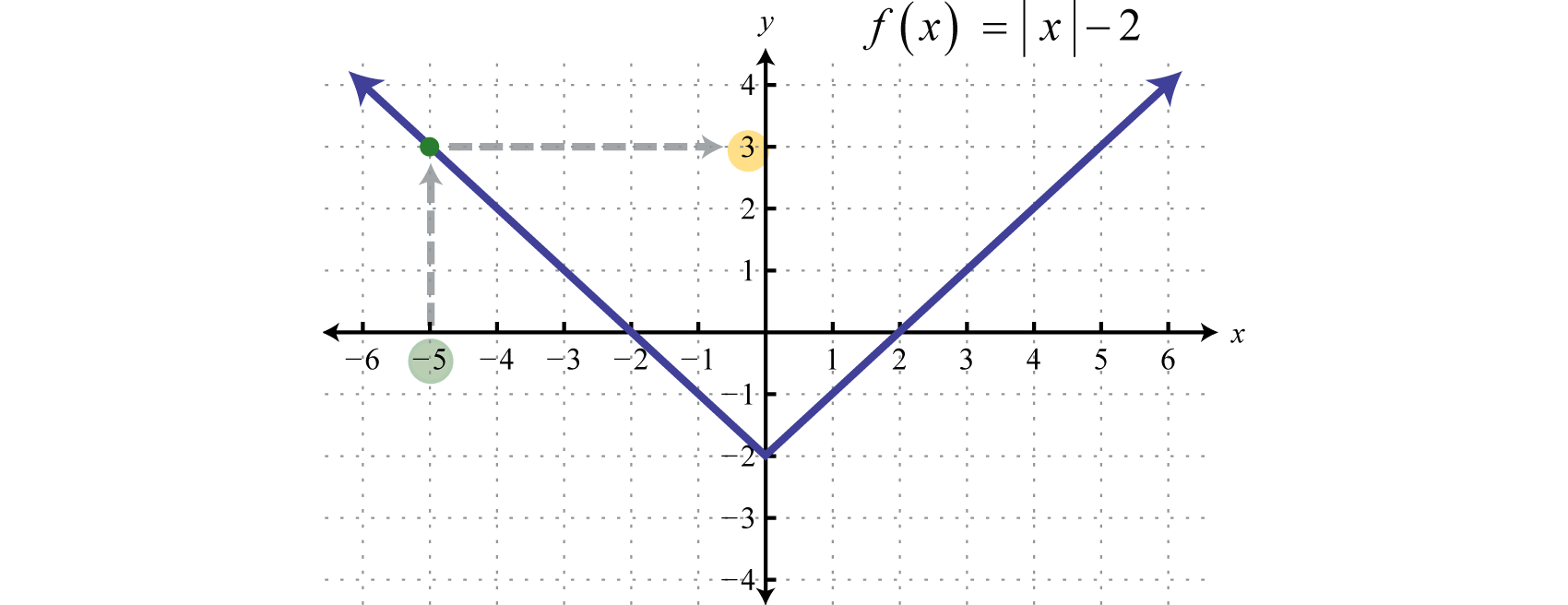Figure 2.1.17

Function notation streamlines the task of evaluating. For example, use the function $$h$$ defined by $$h (x) = \frac{1}{2} x − 3$$to evaluate for $$x$$-values in the set $$\{−2, 0, 7\}$$.

$$\begin{array} { c } { h ( \color{Cerulean}{- 2}\color{Black}{ )} = \frac { 1 } { 2 } ( \color{Cerulean}{- 2}\color{Black}{ )} - 3 = - 1 - 3 = - 4 } \\ { h ( \color{Cerulean}{0}\color{Black}{ )} = \frac { 1 } { 2 } ( \color{Cerulean}{0}\color{Black}{ )} - 3 = 0 - 3 = - 3 } \\ { h ( \color{Cerulean}{7}\color{Black}{ )} = \frac { 1 } { 2 } ( \color{Cerulean}{7}\color{Black}{ )} - 3 = \frac { 7 } { 2 } - 3 = \frac { 1 } { 2 } } \end{array}$$

Given any function defined by $$h(x) = y$$, the value $$x$$ is called the argument of the function17. The argument can be any algebraic expression. For example:

\begin{aligned} h ( \color{Cerulean}{4 a ^ { 3 }}\color{Black}{ )} & = \frac { 1 } { 2 } ( \color{Cerulean}{4 a ^ { 3 } }\color{Black}{)} - 3 = 2 a ^ { 3 } - 3 \\ h ( \color{Cerulean}{2 x - 1}\color{Black}{ )} & = \frac { 1 } { 2 } ( \color{Cerulean}{2 x - 1}\color{Black}{ )} - 3 = x - \frac { 1 } { 2 } - 3 = x - \frac { 7 } { 2 } \end{aligned}

Example $$\PageIndex{5}$$:

Given $$g ( x ) = x ^ { 2 }$$ , find $$g (−2), g ( \frac{1}{2} )$$, and $$g (x + h)$$ .

Solution:

Recall that when evaluating, it is a best practice to begin by replacing the variables with parentheses and then substitute the appropriate values. This helps with the order of operations when simplifying expressions.

\begin{aligned} g ( \color{Cerulean}{- 2}\color{Black}{ )} & = ( \color{Cerulean}{- 2}\color{Black}{ )} ^ { 2 } = 4 \\ g ( \color{Cerulean}{\frac { 1 } { 2 }}\color{Black}{)} & = ( \color{Cerulean}{\frac { 1 } { 2 }} \color{Black}{)} ^ { 2 } = \frac { 1 } { 4 } \\ g (\color{Cerulean}{ x + h}\color{Black}{ )} & = ( \color{Cerulean}{x + h}\color{Black}{ )} ^ { 2 } = x ^ { 2 } + 2 x h + h ^ { 2 } \end{aligned}

$$g (−2) = 4,\: g ( \frac{1}{2} ) = \frac{1}{4} ,\: g (x + h) = x^{2} + 2xh + h^{2}$$

At this point, it is important to note that, in general, $$f (x + h) ≠ f (x) + f (h)$$. The previous example, where $$g (x) = x^{2}$$ , illustrates this nicely

$$\begin{array} { l } { g ( x + h ) \neq g ( x ) + g ( h ) } \\ { ( x + h ) ^ { 2 } \neq x ^ { 2 } + h ^ { 2 } } \end{array}$$

Example $$\PageIndex{6}$$:

Given $$f ( x ) = \sqrt { 2 x + 4 }$$ , find $$f (−2), f (0)$$, and $$f \left( \frac { 1 } { 2 } a ^ { 2 } - 2 \right)$$.

Solution:

\begin{aligned} f ( \color{Cerulean}{- 2}\color{Black}{ )} & = \sqrt { 2 ( \color{Cerulean}{- 2}\color{Black}{ )} + 4 } = \sqrt { - 4 + 4 } = \sqrt { 0 } = 0 \\ f ( \color{Cerulean}{0}\color{Black}{ )} & = \sqrt { 2 ( \color{Cerulean}{0}\color{Black}{ )} + 4 } = \sqrt { 0 + 4 } = \sqrt { 4 } = 2 \\ f ( \color{Cerulean}{\frac { 1 } { 2 } a ^ { 2 } - 2}\color{Black}{ )} & = \sqrt { 2( \color{Cerulean}{ \frac { 1 } { 2 } a ^ { 2 } - 2}\color{Black}{)} + 4 } = \sqrt { a ^ { 2 } - 4 + 4 } = \sqrt { a ^ { 2 } } = | a | \end{aligned}

$$f (−2) = 0,\: f (0) = 2,\: f \left( \frac { 1 } { 2 } a ^ { 2 } - 2 \right)= |a|$$

Example $$\PageIndex{7}$$:

Given the graph of  $$g(x)$$, find $$g(−8), g(0)$$, and $$g(8)$$.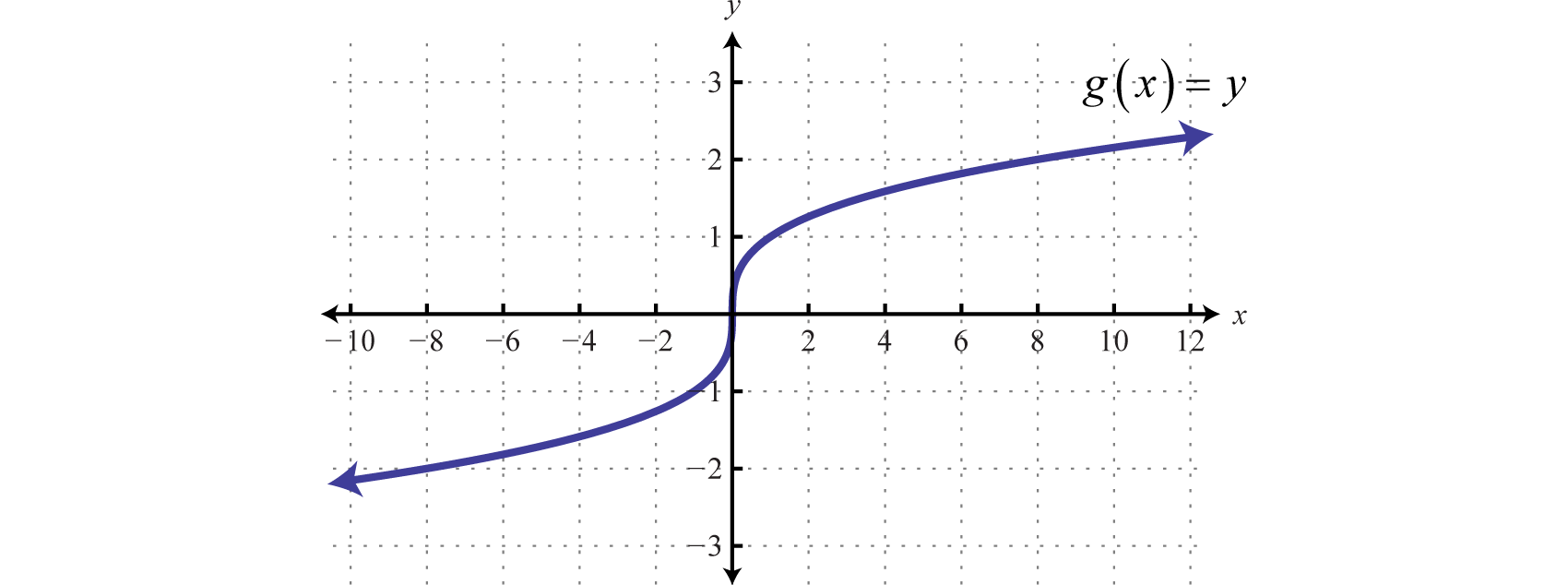Figure 2.1.18

Solution:

Use the graph to find the corresponding $$y$$-values where $$x = −8, 0$$, and $$8$$.Figure 2.1.19

$$g(−8)=−2,\: g(0)=0,\: g(8)=2$$

Sometimes the output is given and we are asked to find the input.

Example $$\PageIndex{8}$$:

Given $$f (x) = 5x + 7$$, find $$x$$ where $$f (x) = 27$$.

Solution:

In this example, the output is given and we are asked to find the input. Substitute $$f (x)$$ with $$27$$ and solve.

$$\begin{array} { c } { f ( x ) = 5 x + 7 }\\\color{Cerulean}{\downarrow}\quad\quad\quad\:\:\: \\ { 27 = 5 x + 7 } \\ { 20 = 5 x } \\ { 4 = x } \end{array}$$

Therefore, $$f (4) = 27$$. As a check, we can evaluate $$f (4) = 5 (4) + 7 = 27$$.

$$x = 4$$

Example $$\PageIndex{9}$$:

Given the graph of $$g$$, find $$x$$ where $$g(x)=2$$.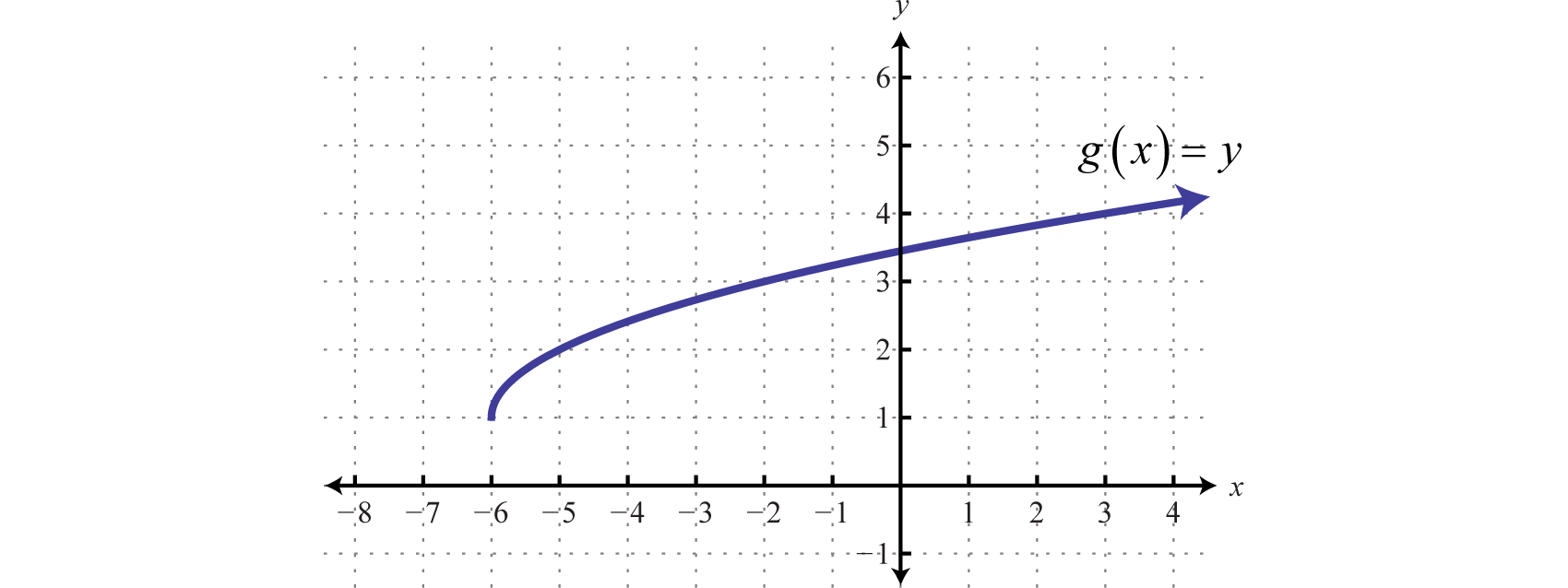Figure 2.1.20

Solution:

Here we are asked to find the x-value given a particular y-value. We begin with 2 on the y-axis and then read the corresponding x-value.Figure 2.1.21

We can see that $$g(x)=2$$ where $$x=−5$$; in other words, $$g(−5)=2$$.

$$x=−5$$

Exercise $$\PageIndex{2}$$

Given the graph of $$h$$, find $$x$$ where $$h(x)=-4$$Figure 2.1.22

$$x=-15$$ and $$x=15$$

Key Takeaways

• A relation is any set of ordered pairs. However, in this course, we will be working with sets of ordered pairs $$(x, y)$$ in the rectangular coordinate system. The set of $$x$$-values defines the domain and the set of $$y$$-values defines the range.
• Special relations where every $$x$$-value (input) corresponds to exactly one $$y$$-value (output) are called functions.
• We can easily determine whether or not an equation represents a function by performing the vertical line test on its graph. If any vertical line intersects the graph more than once, then the graph does not represent a function.
• If an algebraic equation defines a function, then we can use the notation $$f (x) = y$$. The notation $$f (x)$$ is read “f of x” and should not be confused with multiplication. When working with functions, it is important to remember that $$y$$ and $$f (x)$$ are used interchangeably.
• If asked to find $$f(a)$$, we substitute the argument $$a$$ in for the variable and then simplify. The argument could be an algebraic expression.
• If asked to find $$x$$ where $$f(x) = a$$, we set the function equal to $$a$$ and then solve for $$x$$.

Exercise $$\PageIndex{3}$$

Determine the domain and range and state whether the relation is a function or not.

1. $$\{ ( 3,1 ) , ( 5,2 ) , ( 7,3 ) , ( 9,4 ) , ( 12,4 ) \}$$
2. $$\{ ( 2,0 ) , ( 4,3 ) , ( 6,6 ) , ( 8,6 ) , ( 10,9 ) \}$$
3. $$\{ ( 7,5 ) , ( 8,6 ) , ( 10,7 ) , ( 10,8 ) , ( 15,9 ) \}$$
4. $$\{ ( 1,1 ) , ( 2,1 ) , ( 3,1 ) , ( 4,1 ) , ( 5,1 ) \}$$
5. $$\{ ( 5,0 ) , ( 5,2 ) , ( 5,4 ) , ( 5,6 ) , ( 5,8 ) \}$$
6. $$\{ ( - 3,1 ) , ( - 2,2 ) , ( - 1,3 ) , ( 0,4 ) , ( 0,5 ) \}$$Figure 2.1.23

8.Figure 2.1.24

9.Figure 2.1.25

10.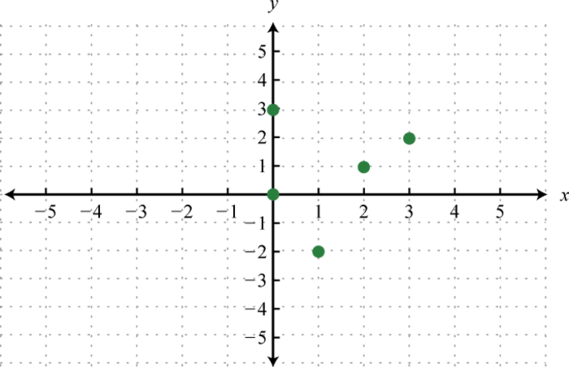Figure 2.1.26

11.Figure 2.1.27

12.Figure 2.1.28

13.Figure 2.1.29

14.Figure 2.1.30

15.Figure 2.1.31

16.Figure 2.1.32

17.Figure 2.1.33

18.Figure 2.1.34

19.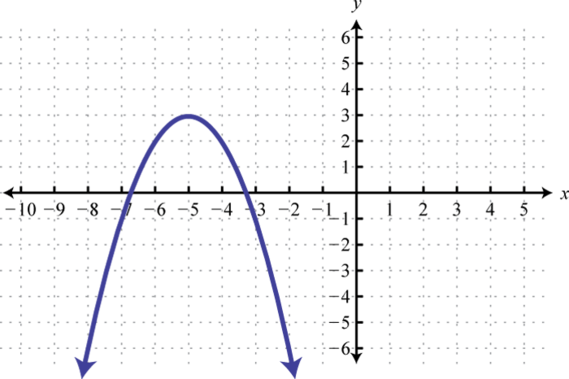Figure 2.1.35

20.Figure 2.1.36

21.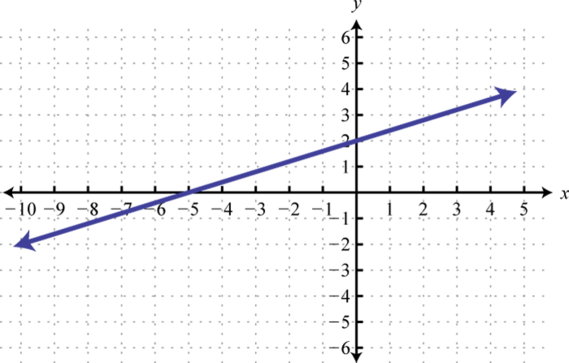Figure 2.1.37

22.Figure 2.1.38

23.Figure 2.1.39

24.Figure 2.1.40

25.Figure 2.1.41

26.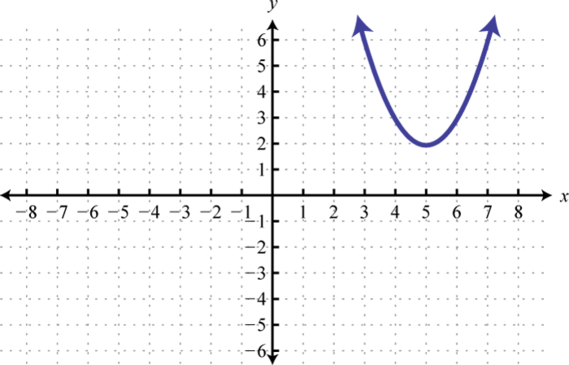Figure 2.1.42

27.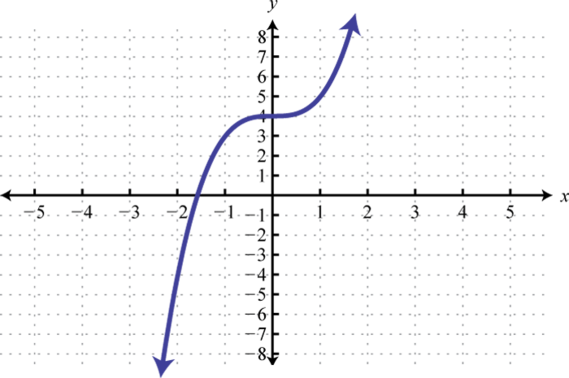Figure 2.1.43

28.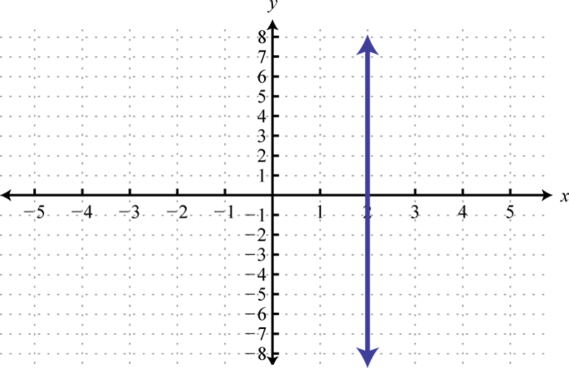Figure 2.1.44

29.Figure 2.1.45

30.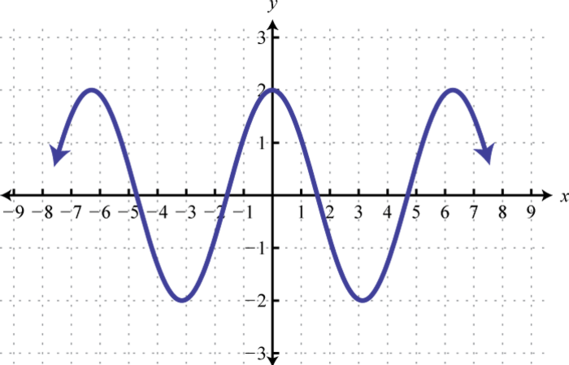Figure 2.1.46

31.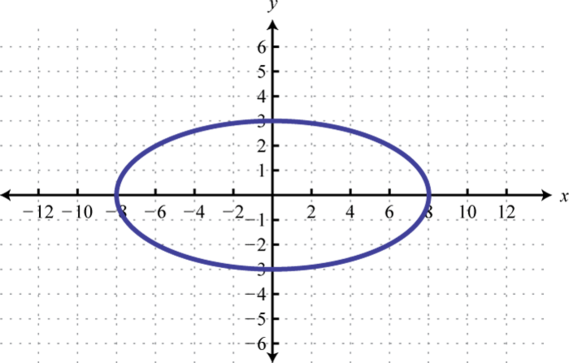Figure 2.1.47

32.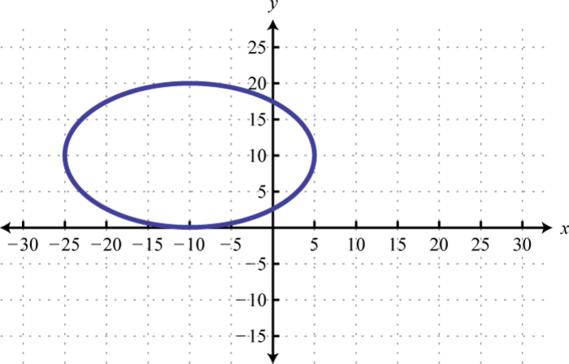Figure 2.1.48

33.Figure 2.1.49

34.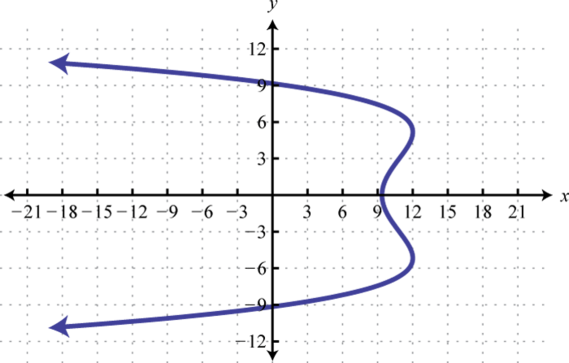Figure 2.1.50

1. Domain: $$\{ 3,5,7,9,12 \}$$; range: $$\{ 1,2,3,4 \}$$; function: yes

3. Domain: $$\{ 7,8,10,15 \}$$; range: $$\{ 5,6,7,8,9 \}$$; function: no

5. Domain: $$\{5\}$$; range: $$\{ 0,2,4,6,8 \}$$; function: no

7. Domain: $$\{ - 4 , - 1,0,2,3 \}$$; range: $$\{ 1,2,3 \}$$; function: yes

9. Domain: $$\{−1, 0, 1, 2\}$$; range: $$\{0, 1, 2, 3, 4\}$$; function: no

11. Domain: $$\{−2\}$$; range: $$\{−4, −2, 0, 2, 4\}$$; function: no

13. Domain: $$ℝ$$; range: $$[−2, ∞)$$; function: yes

15. Domain: $$(−∞, −1]$$; range: $$ℝ$$; function: no

17. Domain: $$(−∞, 0]$$; range: $$[−1, ∞)$$; function: yes

19. Domain: $$ℝ$$; range: $$(−∞, 3]$$; function: yes

21. Domain: $$ℝ$$; range: $$ℝ$$; function: yes

23. Domain: $$[−5, −1]$$; range: $$[−2, 2]$$; function: no

25. Domain: $$ℝ$$; range: $$[0, ∞]$$; function: yes

27. Domain: $$ℝ$$; range: $$ℝ$$; function: yes

29. Domain: $$ℝ$$; range: $$[−1, 1]$$; function: yes

31. Domain: $$[−8, 8]$$; range: $$[−3, 3]$$; function: no

33. Domain: $$ℝ$$; range: $$[−8, ∞]$$; function: yes

Exercise $$\PageIndex{4}$$

Evaluate.

1. $$g ( x ) = | x - 5 | \text { find } g ( - 5 ) , g ( 0 ) , \text { and } g ( 5 )$$
2. $$g ( x ) = | x | - 5 ; \text { find } g ( - 5 ) , g ( 0 ) , \text { and } g ( 5 )$$
3. $$g ( x ) = | 2 x - 3 | ; \text { find } g ( - 1 ) , g ( 0 ) , \text { and } g \left( \frac { 3 } { 2 } \right)$$
4. $$g ( x ) = 3 - | 2 x | ; \text { find } g ( - 3 ) , g ( 0 ) , \text { and } g ( 3 )$$
5. $$f ( x ) = 2 x - 3 ; \text { find } f ( - 2 ) , f ( 0 ) , \text { and } f ( x - 3 )$$
6. $$f ( x ) = 5 x - 1 ; \text { find } f ( - 2 ) , f ( 0 ) , \text { and } f ( x + 1 )$$
7. $$g ( x ) = \frac { 2 } { 3 } x + 1 ; \text { find } g ( - 3 ) , g ( 0 ) , \text { and } f ( 9 x + 6 )$$
8. $$g ( x ) = - \frac { 3 } { 4 } x - \frac { 1 } { 2 } ; \text { find } g ( - 4 ) , g ( 0 ) , \text { and } g ( 6 x - 2 )$$
9. $$g ( x ) = x ^ { 2 } ; \text { find } g ( - 5 ) , g ( \sqrt { 3 } ) , \text { and } g ( x - 5 )$$
10. $$g ( x ) = x ^ { 2 } + 1 ; \text { find } g ( - 1 ) , g ( \sqrt { 6 } ) , \text { and } g ( 2 x - 1 )$$
11. $$f ( x ) = x ^ { 2 } - x - 2 ; \text { find } f ( 0 ) , f ( 2 ) , \text { and } f ( x + 2 )$$
12. $$f ( x ) = - 2 x ^ { 2 } + x - 4 ; \text { find } f ( - 2 ) , f \left( \frac { 1 } { 2 } \right) , \text { and } f ( x - 3 )$$
13. $$h ( t ) = - 16 t ^ { 2 } + 32 ; \text { find } h \left( \frac { 1 } { 4 } \right) , h \left( \frac { 1 } { 2 } \right) , \text { and } h ( 2 a - 1 )$$
14. $$h ( t ) = - 16 t ^ { 2 } + 32 ; \text { find } h ( 0 ) , h ( \sqrt { 2 } ) , h ( 2 a + 1 )$$
15. $$f ( x ) = \sqrt { x + 1 } - 2 \text { find } f ( - 1 ) , f ( 0 ) , f ( x - 1 )$$
16. $$f ( x ) = \sqrt { x - 3 } + 1 ; \text { find } f ( 12 ) , f ( 3 ) , f ( x + 3 )$$
17. $$g ( x ) = \sqrt { x + 8 } ; \text { find } g ( 0 ) , g ( - 8 ) , \text { and } g ( x - 8 )$$
18. $$g ( x ) = \sqrt { 3 x - 1 } ; \text { find } g \left( \frac { 1 } { 3 } \right) , g \left( \frac { 5 } { 3 } \right) , \text { and } g \left( \frac { 1 } { 3 } a ^ { 2 } + \frac { 1 } { 3 } \right)$$
19. $$f ( x ) = x ^ { 3 } + 1 ; \text { find } f ( - 1 ) , f ( 0 ) , f \left( a ^ { 2 } \right)$$
20. $$f ( x ) = x ^ { 3 } - 8 ; \text { find } f ( 2 ) , f ( 0 ) , f \left( a ^ { 3 } \right)$$

1. $$g ( - 5 ) = 10 , g ( 0 ) = 5 , g ( 5 ) = 0$$

3. $$g ( - 1 ) = 5 , g ( 0 ) = 3 , g \left( \frac { 3 } { 2 } \right) = 0$$

5. $$f ( - 2 ) = - 7 , f ( 0 ) = - 3 , f ( x - 3 ) = 2 x - 9$$

7. $$g ( - 3 ) = - 1 , g ( 0 ) = 1 , g ( 9 x + 6 ) = 6 x + 5$$

9. $$g ( - 5 ) = 25 , g ( \sqrt { 3 } ) = 3 , g ( x - 5 ) = x ^ { 2 } - 10 x + 25$$

11. $$f ( 0 ) = - 2 , f ( 2 ) = 0 , f ( x + 2 ) = x ^ { 2 } + 3 x$$

13. $$h \left( \frac { 1 } { 4 } \right) = 31 , h \left( \frac { 1 } { 2 } \right) = 28 , h ( 2 a - 1 ) = - 64 a ^ { 2 } + 64 a + 16$$

15. $$f ( - 1 ) = - 2 , f ( 0 ) = - 1 , f ( x - 1 ) = \sqrt { x } - 2$$

17. $$g ( 0 ) = 2 \sqrt { 2 } , g ( - 8 ) = 0 , g \left( a ^ { 2 } - 8 \right) = | a |$$

19. $$f ( - 1 ) = 0 , f ( 0 ) = 1 , f \left( a ^ { 2 } \right) = a ^ { 6 } + 1$$

Exercise $$\PageIndex{5}$$

Given the function find $$f(x+h)$$.

1. $$f ( x ) = 3 x - 1$$
2. $$f ( x ) = - 5 x + 2$$
3. $$f ( x ) = x ^ { 2 } + x + 1$$
4. $$f ( x ) = 2 x ^ { 2 } - x - 1$$
5. $$f ( x ) = x ^ { 3 }$$
6. $$f ( x ) = 2 x ^ { 3 } - 1$$

1. $$f ( x + h ) = 3 x + 3 h - 1$$

3. $$f ( x + h ) = x ^ { 2 } + 2 x h + h ^ { 2 } + x + h + 1$$

5. $$f ( x + h ) = x ^ { 3 } + 3 h x ^ { 2 } + 3 h ^ { 2 } x + h ^ { 3 }$$

Exercise $$\PageIndex{6}$$

Find $$x$$ given the function.

1. $$f ( x ) = 2 x - 3 ; \text { find } x \text { where } f ( x ) = 25$$
2. $$f ( x ) = 7 - 3 x ; \text { find } x \text { where } f ( x ) = - 27$$
3. $$f ( x ) = 2 x + 5 ; \text { find } x \text { where } f ( x ) = 0$$
4. $$f ( x ) = - 2 x + 1 ; \text { find } x \text { where } f ( x ) = 0$$
5. $$g ( x ) = 6 x + 2 ; \text { find } x \text { where } g ( x ) = 5$$
6. $$g ( x ) = 4 x + 5 ; \text { find } x \text { where } g ( x ) = 2$$
7. $$h ( x ) = \frac { 2 } { 3 } x - \frac { 1 } { 2 } ; \text { find } x \text { where } h ( x ) = \frac { 1 } { 6 }$$
8. $$h ( x ) = \frac { 5 } { 4 } x + \frac { 1 } { 3 } ; \text { find } x \text { where } h ( x ) = \frac { 1 } { 2 }$$
9. The value of a new car in dollars is given by the function $$V(t) = −1,800t + 22,000$$ where $$t$$ represents the age of the car in years. Use the function to determine the value of the car when it is $$4$$ years old. What was the value of the car new?
10. The monthly income in dollars of a commissioned car salesperson is given by the function $$I(n) = 350n + 1,450$$ where $$n$$ represents the number of cars sold in the month. Use the function to determine the salesperson’s income if he sells $$3$$ cars this month. What is his income if he does not sell any cars in one month?

1. $$x=14$$

3. $$x=-\frac{5}{2}$$

5. $$x=\frac{1}{2}$$

7. $$x=1$$

9. New: $$22,000$$; $$4$$ years old: $$14,800$$

Exercise $$\PageIndex{7}$$

Given the graph of the function $$f$$, find the function values.

1. Find $$f ( 0 ) , f ( 2 )$$ , and $$f ( 4 )$$.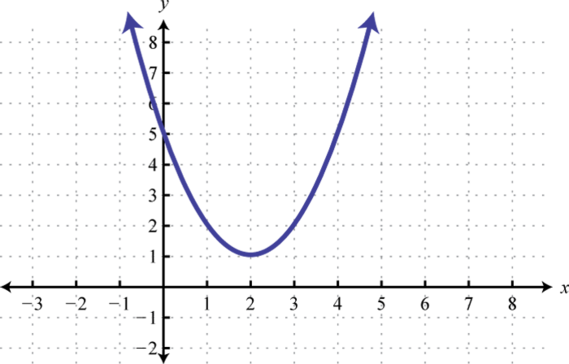Figure 2.1.51

2. Find $$f(-1), f(0)$$, and $$f(1)$$.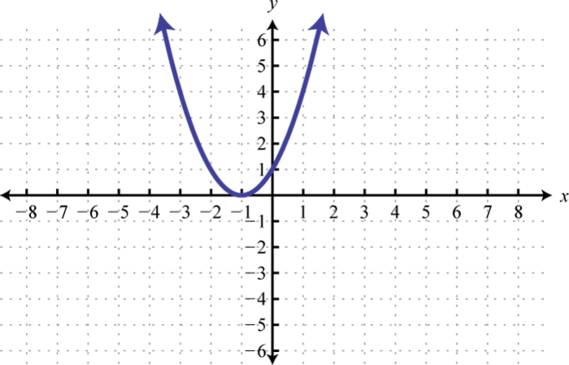Figure 2.1.52

3. Find $$f (0), f (2)$$, and $$f (4)$$.Figure 2.1.53

4. Find $$f (−3), f (0)$$, and $$f (3)$$.Figure 2.1.54

5. Find $$f (−4), f (0)$$, and $$f (2)$$.\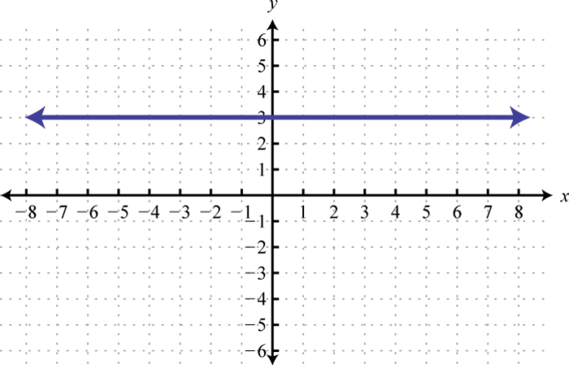Figure 2.1.55

6. Find $$f (−6), f (0)$$, and $$f (6)$$.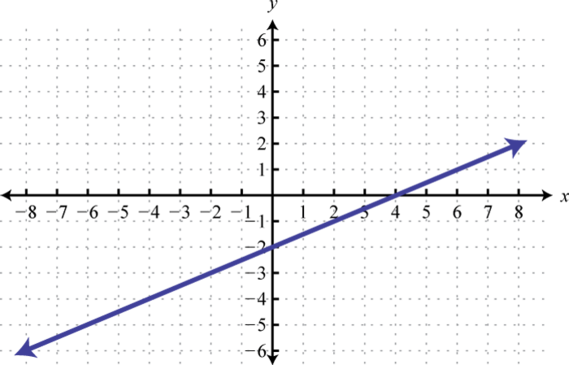Figure 2.1.56

7. Find $$f (−2), f (2)$$, and $$f (7)$$.Figure 2.1.57

8. Find $$f (0), f (5)$$, and $$f (9)$$.Figure 2.1.58

9. Find $$f (−8), f (0)$$, and $$f (8)$$.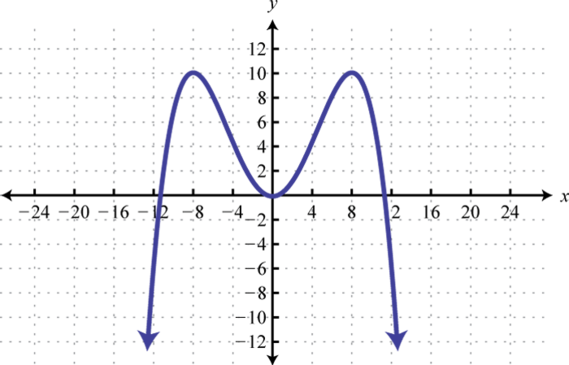Figure 2.1.59

10. Find $$f (−12), f (0)$$, and $$f (12)$$.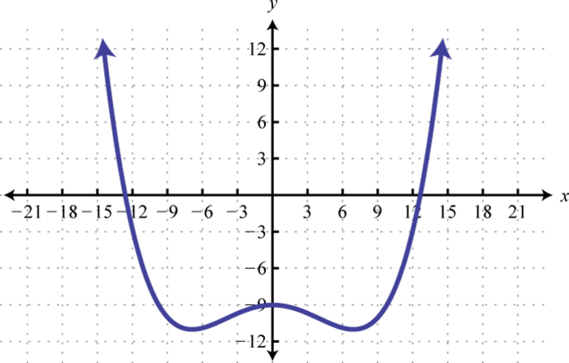Figure 2.1.60

1. $$f (0) = 5, f (2) = 1, f (4) = 5$$

3. $$f (0) = 0, f (2) = 2, f (4) = 0$$

5. $$f (−4) = 3, f (0) = 3, f (2) = 3$$

7. $$f (−2) = 1, f (2) = 3, f (7) = 4$$

9. $$f (−8) = 10, f (0) = 0, f (8) = 10$$

Exercise $$\PageIndex{8}$$

Given the graph of a function $$g$$, find the $$x$$-values:

1. Find $$x$$ where $$g (x) = 3, g (x) = 0$$, and $$g (x) = −2$$.Figure 2.1.61

2. Find $$x$$ where $$g (x) = 0, g (x) = 1$$, and $$g (x) = 4$$.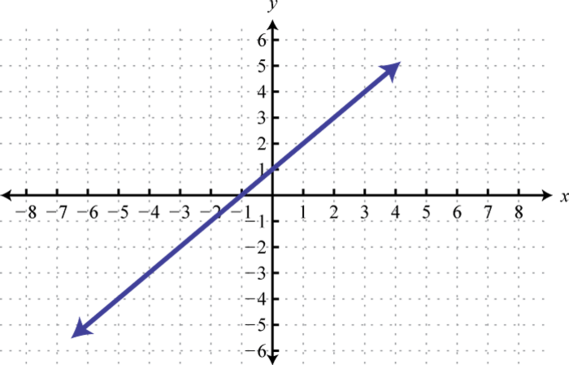Figure 2.1.62

3. Find $$x$$ where $$g (x) = −5, g (x) = 0$$, and $$g (x) = 10$$.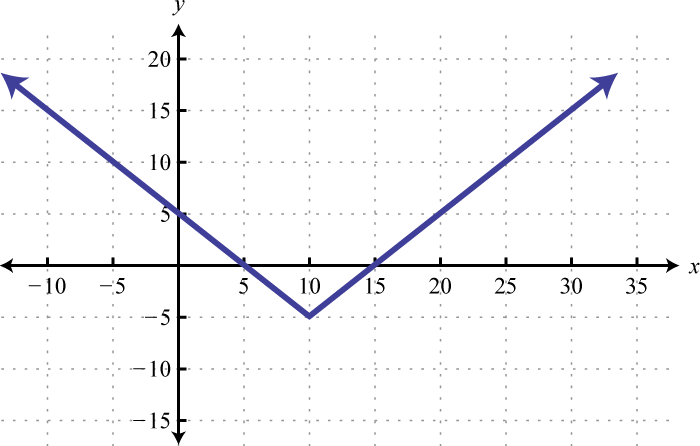Figure 2.1.63

4. Find $$x$$ where $$g (x) = 0, g (x) = 10$$, and $$g (x) = 15$$.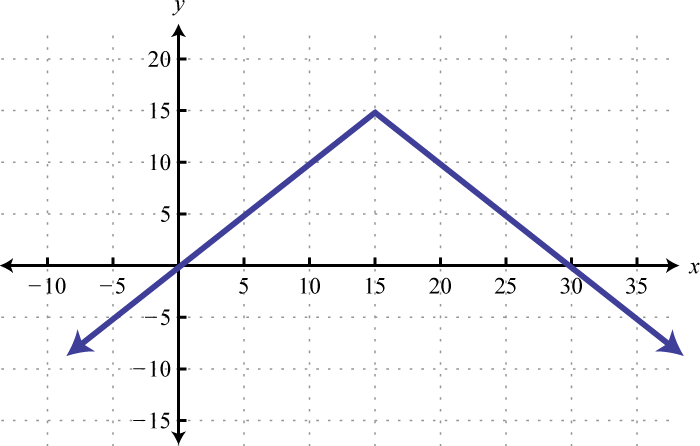Figure 2.1.64

5. Find $$x$$ where $$g (x) = −5, g (x) = −4$$, and $$g (x) = 4$$.\Figure 2.1.65

6. Find $$x$$ where $$g (x) = 1, g (x) = 0$$, and $$g (x) = −3$$.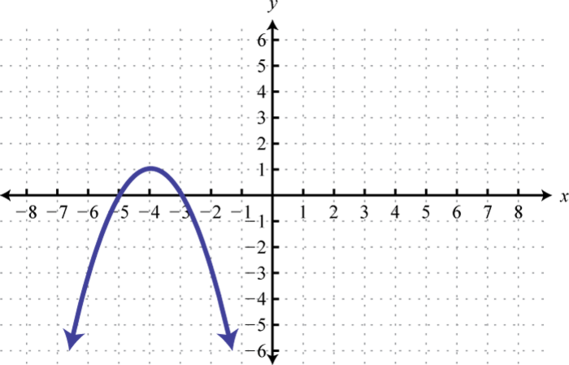Figure 2.1.66

7. Find $$x$$ where $$g (x) = −4, g (x) = 3$$, and $$g (x) = 4$$.Figure 2.1.67

8. Find $$x$$ where $$g (x) = −5, g (x) = −4$$, and $$g (x) = 4$$.Figure 2.1.68

9. Find $$x$$ where $$g (x) = −10$$ and $$g (x) = 5$$.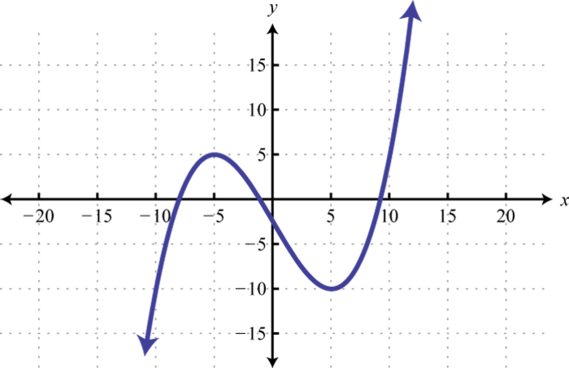Figure 2.1.69

10. Find $$x$$ where $$g(x)=2$$.Figure 2.1.70

The value of a certain automobile in dollars depends on the number of years since it was purchased in $$1970$$ according to the following function:Figure 2.1.71

11. What was the value of the car when it was new in $$1970$$?

12. In what year was the value of the car at a minimum?

13. What was the value of the car in $$2005$$?

14. In what years was the car valued at $$4,000$$?

1. $$g (−4) = 3, g (2) = 0$$, and $$g (6) = −2$$.

3. $$g (10) = −5, g (5) = 0$$ and $$g (15) = 0 , g (−5) = 10$$ and $$g (25) = 10$$

5. $$g (−2) = −5, g (−3) = −4$$ and $$g (−1) = −4 , g (−5) = 4$$ and $$g (1) = 4$$

7. $$g (−2) = −4, g (−1) = 3, g (0) = 4$$

9. $$g (−10) = −10$$ and $$g (5) = −10 ; g (−5) = 5$$ and $$g (10) = 5$$

11. $$5,000$$

13. $$10,000$$

Exercise $$\PageIndex{9}$$

Given the linear function defined by $$f(x)=2x-5$$, simplify the following.

1. $$f ( 5 ) - f ( 3 )$$
2. $$f ( 0 ) - f ( 7 )$$
3. $$f ( x + 2 ) - f ( 2 )$$
4. $$f ( x + 7 ) - f ( 7 )$$
5. $$f ( x + h ) - f ( x )$$
6. $$\frac { f ( x + h ) - f ( x ) } { h }$$
7. Simplify $$\frac { c ( x + h ) - c ( x ) } { h }$$ given $$c ( x ) = 3 x + 1$$.
8. Simplify $$\frac { p ( x + h ) - p ( x ) } { h }$$ given $$p ( x ) = 7 x - 3$$.
9. Simplify $$\frac { g ( x + h ) - g ( x ) } { h }$$ given $$g ( x ) = m x + b$$.
10. Simplify $$\frac { q ( x + h ) - q ( x ) } { h }$$ given $$q ( x ) = a x$$.

1. $$4$$

3. $$2x$$

5. $$2h$$

7. $$3$$

9. $$m$$

Exercise $$\PageIndex{10}$$

1. Who is credited with the introduction of the notation $$y = f (x)$$? Provide a brief summary of his life and accomplishments.
2. Explain to a beginning algebra student what the vertical line test is and why it works.
3. Research and discuss the life and contributions of René Descartes.
4. Conduct an Internet search for the vertical line test, functions, and evaluating functions. Share a link to a page that you think others may find useful.

Footnotes

1A system with two number lines at right angles specifying points in a plane using ordered pairs $$(x, y)$$.

2The horizontal number line used as reference in a rectangular coordinate system.

3The vertical number line used as reference in a rectangular coordinate system.

4The flat surface defined by $$x$$- and $$y$$-axes.

5Pairs $$(x, y)$$ that identify position relative to the origin on a rectangular coordinate plane.

6The point where the $$x$$- and $$y$$-axes cross, denoted by $$(0, 0)$$.

7The four regions of a rectangular coordinate plane partly bounded by the $$x$$- and $$y$$-axes and numbered using the Roman numerals I, II, III, and IV.

8Term used in honor of René Descartes when referring to the rectangular coordinate system.

9Any set of ordered pairs.

10A visual representation of a relation on a rectangular coordinate plane.

11The set consisting of all of the first components of a relation. For relations consisting of points in the plane, the domain is the set of all $$x$$-values.

12The set consisting of all of the second components of a relation. For relations consisting of points in the plane, the range is the set of all $$y$$-values.

13Used when referencing the range.

14A relation where each element in the domain corresponds to exactly one element in the range.

15If any vertical line intersects the graph more than once, then the graph does not represent a function.

16The notation $$f (x) = y$$ , which reads “$$f$$ of $$x$$ is equal to $$y$$.” Given a function, $$y$$ and $$f (x)$$ can be used interchangeably.

17The value or algebraic expression used as input when using function notation.